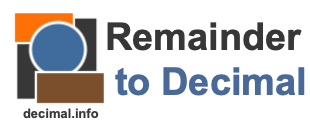Remainder to Decimal CalculatorThe Remainder to Decimal Calculator is helpful if you solved a division problem and your answer had a remainder. This calculator will convert your long division answer with a remainder (R) to a decimal number.